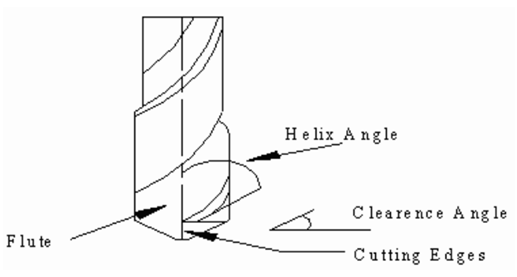## Drilling Assignment Help

Drilling

It is carried out on drilling machine as well as on the lathe. Commonly Twist drill is utilized for drilling operations. Drilling is the operation from which holes are generated. Essential feature of drilling is the variation in cutting speed along the cutting edge. At the periphery the speed is maximum that produced cylindrical surface and approaches zero near center-line of drill. There are only even numbers of tooth on twist drill for balancing purpose. Figure illustrated the nomenclature of twist drills.Figure: Twist Drill

In the drilling the cutting speed is given by following equation

V = π DN /1000                           eq. (1)

Where,

V = cutting speed, m/min

D = diameter of the twist drill, mm

N = rotational speed of the drill, rev/min

The drill ought to approach the start of the hole from a distance say, AI, and also traverse beyond the actual hole by a distance called as the total approach allowance (A). In general, AI is taken as 2 to 3 mm. Total approach distance is given by

A = (D /2) tan ϒ                          eq. (2)

Where,  ϒ = 59o in common case

So,  A = D /3.3286

Total length of tool travel

L = (l + A + 2) mm                       eq.(3)

Where, l = length of hole

Therefore, time for drilling the hole =

L /( f * N )                                    eq.(4)

Where, f = feed rate in mm/rev.